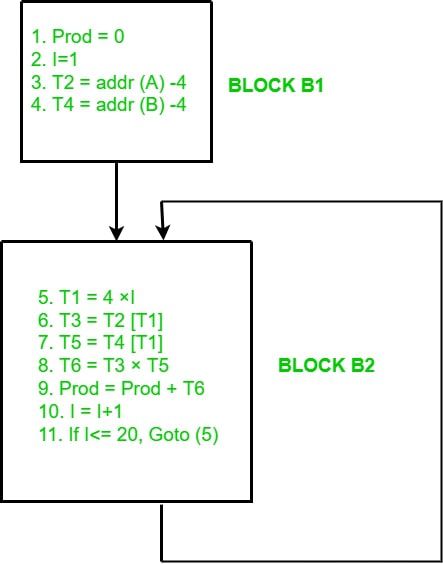Open in App
Not now

# Flow Graph in Code Generation

• Difficulty Level : Medium
• Last Updated : 06 Mar, 2022

A basic block is a simple combination of statements. Except for entry and exit, the basic blocks do not have any branches like in and out. It means that the flow of control enters at the beginning and it always leaves at the end without any halt. The execution of a set of instructions of a basic block always takes place in the form of a sequence.

The first step is to divide a group of three-address codes into the basic block. The new basic block always begins with the first instruction and continues to add instructions until it reaches a jump or a label. If no jumps or labels are identified, the control will flow from one instruction to the next in sequential order.

The algorithm for the construction of the basic block is described below step by step:

Algorithm: The algorithm used here is partitioning the three-address code into basic blocks.

Input: A sequence of three-address codes will be the input for the basic blocks.

Output: A list of basic blocks with each three address statements, in exactly one block, is considered as the output.

Method: We’ll start by identifying the intermediate code’s leaders. The following are some guidelines for identifying leaders:

1. The first instruction in the intermediate code is generally considered as a leader.
2. The instructions that target a conditional or unconditional jump statement can be considered as a leader.
3. Any instructions that are just after a conditional or unconditional jump statement can be considered as a leader.

Each leader’s basic block will contain all of the instructions from the leader until the instruction right before the following leader’s start.

Example of basic block:

Three Address Code for the expression a = b + c – d is:

```T1 = b + c
T2 = T1 - d
a = T2```

This represents a basic block in which all the statements execute in a sequence one after the other.

### Basic Block Construction:

Let us understand the construction of basic blocks with an example:

Example:

```1. PROD = 0
2. I = 1
3. T2 = addr(A) – 4
4. T4 = addr(B) – 4
5. T1 = 4 x I
6. T3 = T2[T1]
7. T5 = T4[T1]
8. T6 = T3 x T5
9. PROD = PROD + T6
10. I = I + 1
11. IF I <=20 GOTO (5)```

Using the algorithm given above, we can identify the number of basic blocks in the above three-address code easily-

There are two Basic Blocks in the above three-address code:

• B1 – Statement 1 to 4
• B2 – Statement 5 to 11

### Transformations on Basic blocks:

Transformations on basic blocks can be applied to a basic block. While transformation, we don’t need to change the set of expressions computed by the block.

There are two types of basic block transformations. These are as follows:

### 1. Structure-Preserving Transformations

Structure preserving transformations can be achieved by the following methods:

1. Common sub-expression elimination
3. Renaming of temporary variables
4. Interchange of two independent adjacent statements

### 2. Algebraic Transformations

In the case of algebraic transformation, we basically change the set of expressions into an algebraically equivalent set.

For example, and expression

```x:= x + 0
or x:= x *1 ```

This can be eliminated from a basic block without changing the set of expressions.

### Flow Graph:

A flow graph is simply a directed graph. For the set of basic blocks, a flow graph shows the flow of control information. A control flow graph is used to depict how the program control is being parsed among the blocks. A flow graph is used to illustrate the flow of control between basic blocks once an intermediate code has been partitioned into basic blocks. When the beginning instruction of the Y block follows the last instruction of the X block, an edge might flow from one block X to another block Y.

Let’s make the flow graph of the example that we used for basic block formation:Flow Graph for above Example

Firstly, we compute the basic blocks (which is already done above). Secondly, we assign the flow control information.

My Personal Notes arrow_drop_up
Related Articles